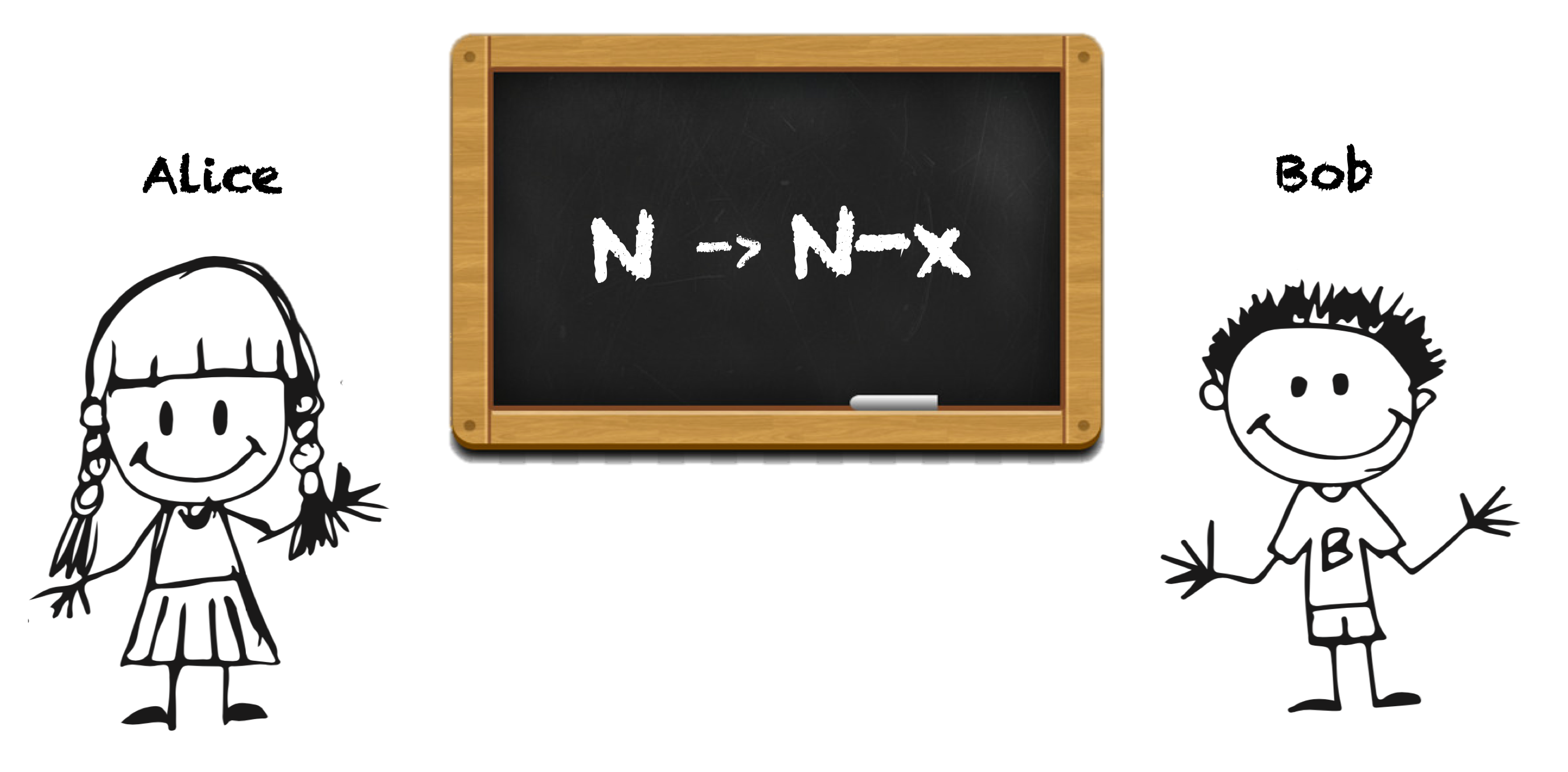# 1025. Divisor Game (C++)

## Problem Setting¶

Alice and Bob take turns playing a game, with Alice starting first.

Initially, there is a number N on the chalkboard. On each player's turn, that player makes a move consisting of:

Choosing any x with 0 < x < N and N % x == 0. Replacing the number N on the chalkboard with N - x. Also, if a player cannot make a move, they lose the game.

Return True if and only if Alice wins the game, assuming both players play optimally.### Example 1:¶

Input: 2

Output: true

Explanation: Alice chooses 1, and Bob has no more moves.

### Example 2:¶

Input: 3

Output: false

Explanation: Alice chooses 1, Bob chooses 1, and Alice has no more moves.

Note: 1 <= N <= 1000

## Solution from Ho Ngan Hang¶

In :
#include <iostream>
using namespace std;

class Solution {
public:
bool divisorGame(int N) {
vector<bool> v(N + 1);
for (int i = 0; i < N; i++) {
if (!v[i]) {
for (int j = 1; i + j <= N && j < i + j; j++)
if ((i + j) % j == 0)
v[i + j] = 1;
}
}
return v[N];
}
};

In :
Solution S;
// Example 1
S.divisorGame(2)

Out:
true
In :
// Example 2
S.divisorGame(3)

Out:
false

## Step-by-step understanding¶

// Step. 1
// - Define the function with input integer N
// - this returns N's element
bool divisorGame(int N) {
// ...
}
return v[N];

// Step. 2
// initialize boolean array of N + 1
vector<bool> v(N + 1);

// Step. 3
// - for loop for i from 0 to N-1
for (int i = 0; i < N; i++) {
...
}

// Step. 4
// - if i's element is not null, which means it is not calclated be fore
if (!v[i]) {
}

// Step. 5
// - another for loop for j from 1 to i+j
for (int j = 1; i + j <= N && j < i + j; j++)

// Step. 6
// if i + j can be divided by j i.e., j is divisor for i + j
if ((i + j) % j == 0)

// Step. 7
// Insert 1 at i+j's element in v
v[i + j] = 1;